## How to Calculate Age in the Google Sheets using the Date of Birth? (with Example)

Have you ever wondered about calculating age using the date of birth in Google Sheets?. If yes, then you are right! With Google Sheets, you can determine the current time, generate charts, and calculate age based on a birthdate. To calculate the age in google sheets, we can use the DATEDIF function. In this article, let’s discuss how to calculate the age using the date of birth with the help of Google Sheets tips provided on this page.

## Calculate the Age in Google Sheets

Google Sheets calculate the age by using the user’s date of birth and the current date.

You can calculate the age in any of the below two methods,

• The total number of years only
• The total number of years, months, and days

Let’s discuss on above in detail.

### Calculate Age in Google Sheets – Years only

To Calculate the Age with years only, you need to specify today’s date and date of birth.

Formula:

=DATEDIF(dob,curr_date,”Y”)

In the above formula,

dob – The Date of birth mentioned with date, month, and year

curr_date – This is the current date i.e., today’s date to calculate the age

“Y” – This represents the Year. The result will be returned in Years only.

Example: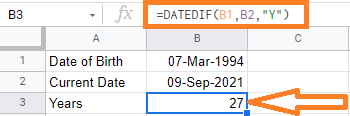In the above example, we are finding the age of the date of birth – 07-Mar-1994.

Cell B1 is having the date of birth and the B2 cell is having the current date. With the expression =DATEDIF(B1, B2, “Y”) we have got 27 years

For getting the Current date, we could also use the TODAY() function in Google Sheets. i.e.,

=DATEDIF(B1,TODAY(), “Y”)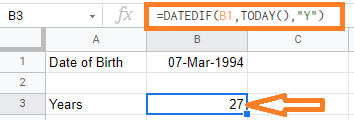In the above image, you could see we are using the TODAY() function to get today’s date.

### Calculate Age in Google Sheets – Years, Months, and Days

To calculate the number of years, months and days, then we need to expand our DATTEDIF Formula.

Let’s first see how to get the individual parts of the result (i.e., the year, month, and day value).

The below formula would give you the year value:

=DATEDIF(dob,curr_date,”Y”)

In the above formula,

dob – The Date of birth mentioned with date, month, and year.

curr_date – This is the current date i.e., today’s date to calculate the age.

“Y” – This represents the Year. The result will be returned in Years only.

Example:In the above example, we are finding the age for the date of birth – 07-Mar-1994.

Cell B1 is having the date of birth and the B2 cell is having the current date. With the expression =DATEDIF(B1, B2, “Y”) we have got 27 years.

The below formula would give you the month value,

=DATEDIF(dob,curr_date,”YM”)

In the above formula,

dob – The Date of birth mentioned with date, month, and year.

curr_date – This is the current date i.e., today’s date to calculate the age.

“YM” – This represents the Month. The result will be returned in Months only.

Example: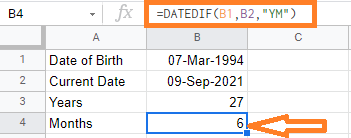In the above example, we are finding the current age month of the date of birth – 07-Mar-1994.

Cell B1 is having the date of birth and the B2 cell is having the current date. With the expression =DATEDIF(B1, B2, “YM”) we have got 6 months.

The below formula would give you the day value,

=DATEDIF(dob,curr_date,”MD”)

In the above formula,

dob – The Date of birth mentioned with date, month, and year

curr_date – This is the current date i.e., today’s date to calculate the age

“MD” – This represents the Day value. The result will be returned in Days only.

Example: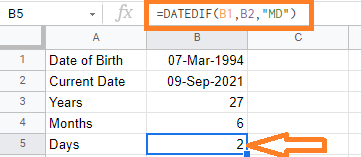In the above example, we are finding the current days difference for the date of birth – 07-Mar-1994.

Cell B1 is having the date of birth and the B2 cell is having the current date. With the expression =DATEDIF(B1, B2, “MD”) we have got 2 days.

The below formula would give you a year, month, and day value

=DATEDIF(B1,B2,“Y”)&” Years “&DATEDIF(B1,B2,“YM”)&” Months & “&DATEDIF(B1,B2,“MD”)&” Days”

In the above formula,

we are concatenating the years, months, and days with the “&” symbol.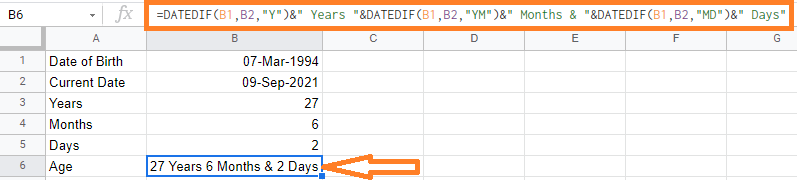You can use the above formula for not only calculating the age but to find the project duration, Employee joining date, and retirement date, and many more.